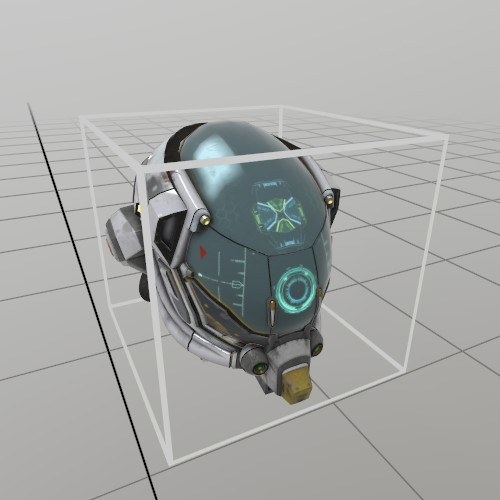# struct Bounds

Bounds is an axis aligned bounding box type that can be used for storing the sizes of objects, calculating containment, intersections, and more!

While the constructor uses a center+dimensions for creating a bounds, don’t forget the static From* methods that allow you to define a Bounds from different types of data!

## Instance Fields and Properties

Vec3 center The exact center of the Bounds!
Vec3 dimensions The total size of the box, from one end to the other. This is the width, height, and depth of the Bounds.

## Instance Methods

Bounds Creates a bounding box object!
Contains Does the Bounds contain the given point? This includes points that are on the surface of the Bounds.
Intersect Calculate the intersection between a Ray, and these bounds. Returns false if no intersection occurred, and ‘at’ will contain the nearest intersection point to the start of the ray if an intersection is found!
Scale Scale this bounds. It will scale the center as well as the dimensions! Modifies this bounds object.
Scaled Scale the bounds. It will scale the center as well as the dimensions! Returns a new Bounds.

## Static Methods

FromCorner Create a bounding box from a corner, plus box dimensions.
FromCorners Create a bounding box between two corner points.

## Examples

### General Usage

``````// All these create bounds for a 1x1x1m cube around the origin!
Bounds bounds = new Bounds(Vec3.One);
bounds = Bounds.FromCorner(new Vec3(-0.5f, -0.5f, -0.5f), Vec3.One);
bounds = Bounds.FromCorners(
new Vec3(-0.5f, -0.5f, -0.5f),
new Vec3( 0.5f,  0.5f,  0.5f));

// Note that positions must be in a coordinate space relative to
// the bounds!
if (bounds.Contains(new Vec3(0,0.25f,0)))
Log.Info("Super easy to check if something's in it!");

// Casting a ray at a bounds is trivial too, again, ensure
// coordinates are in the same space!
Ray ray = new Ray(Vec3.Up, -Vec3.Up);
if (bounds.Intersect(ray, out Vec3 at))
Log.Info("Bounds intersection at " + at); // <0, 0.5f, 0>

// You can also scale a Bounds using the '*' operator overload,
// this is really useful if you're working with the Bounds of a
// Model that you've scaled. It will scale the center as well as
// the size!
bounds = bounds * 0.5f;

// Scale the current bounds reference using 'Scale'
bounds.Scale(0.5f);

// Scale the bounds by a Vec3
bounds = bounds * new Vec3(1, 10, 0.5f);
``````

### An Interactive ModelIf you want to grab a Model and move it around, then you can use a `UI.Handle` to do it! Here’s an example of loading a GLTF from file, and using its information to create a Handle and a UI ‘cage’ box that indicates an interactive element.

``````Model model      = Model.FromFile("DamagedHelmet.gltf");
Pose  handlePose = new Pose(0,0,0, Quat.Identity);
float scale      = .15f;

public void Step() {
UI.HandleBegin("Model Handle", ref handlePose, model.Bounds*scale);

model.Draw(Matrix.S(scale));
Mesh.Cube.Draw(Material.UIBox, Matrix.TS(model.Bounds.center*scale, model.Bounds.dimensions*scale));

UI.HandleEnd();
}
``````

Found an issue with these docs, or have some additional questions? Create an Issue on Github!